Math Calculators
Square Footage Calculator

# Square Footage Calculator

Square footage calculator finds the surface area (square footage) of various shapes and calculates associated construction costs. It can be used as a room area calculator.

Options

Result
Square Footage 5.0106 ft²
Square Inches 721.52644 in²
Square Yards 0.55673 yd²
Square Meters 0.4655
Acres 0.00012 acre
Hectares 0.00005 ha
Cost $7215.2644 There was an error with your calculation. Ads by Google ## Table of Contents## Square footage calculator This square footage calculator is an online tool that calculates the surface areas of different geometrical shapes. The shapes include square, triangle, circle, rectangle, rectangle border, circle border, annulus, and trapezoid. The calculator allows calculating square footage for any of the shapes listed above, which are the most common shapes used during construction. This square footage calculator is handy for finding the surface area of a room or a garden and estimating how much material you would require for construction. The calculator also estimates the necessary costs for construction or renovation (if the price per square unit is known). The primary units of the calculator are square feet. However, the answer is also given in square inches, square yards, square meters, and acres. ## Application Instructions To use the calculator, first, choose the necessary shape from the drop-down list at the top. Then input the required values into the corresponding fields and select the units for each value. Next, choose the quantity of the shapes—for example, if you have two rooms with the same length and width, you can enter the dimensions for one room and input "2" in the "Quantity" field. The calculator will automatically multiply the resulting answer by two, giving you the surface area of both rooms. If you only need the surface area, you can skip the "Optional cost calculation" and immediately press "Calculate." The calculator will return the chosen shape's square footage and surface area value in different units. If you would like to calculate the related costs—for example, the cost of covering the floor with the tiles—enter the price per square unit of the desired material. The supported units for cost calculation are square inches, square feet, square yards, and square meters. Then press "Calculate." The calculator will return the value of the surface area and the costs associated with covering this area with the needed material. ## Important notes about the units The calculator takes only one unit at a time. To convert inches to feet, divide the value in inches by 12. For example, if your measured length is 5 ft 3 in, you can input it as 5.25 ft, since 3 in = 3/12 ft = 1/4 ft = 0.25 ft. Alternatively, you can insert the same value as 63 in, since 5 ft = 5 × 12 in = 60 in. Similarly, in metric units, if you have a length of 3 m 60 cm, you can either input it as 3.6 m or 36 cm. As mentioned above, the primary units of the calculator are feet (and square feet). However, you can input the given values in inches, feet, yards, millimeters, centimeters, and meters. Note that to convert millimeters to feet, you need to divide the value by 304.8. This means that 1 mm = 0.00328084 ft. Suppose the inserted values in millimeters are too small. In that case, the calculator will not be able to provide the answer since the value will be too close to zero. ## Unit conversion rules The calculator uses the following unit conversion rules: • Square feet to square inches: multiply the value in square feet by 144 to get the value in square inches. To convert square inches to square feet, divide the value in square inches by 144. • Square feet to square yards: divide the value in square feet by 9 to get the value in square yards. • Square feet to square meters: divide the value in square feet by 10,764 to get the value in square meters. • Square feet to acre: divide the value in square feet by 43,560 to get the value in an acre. ## Area shapes and formulas The formulas for all the shapes used by the calculator are presented below. In all formulas, A is the area (square footage). All other notations are described below. ## Square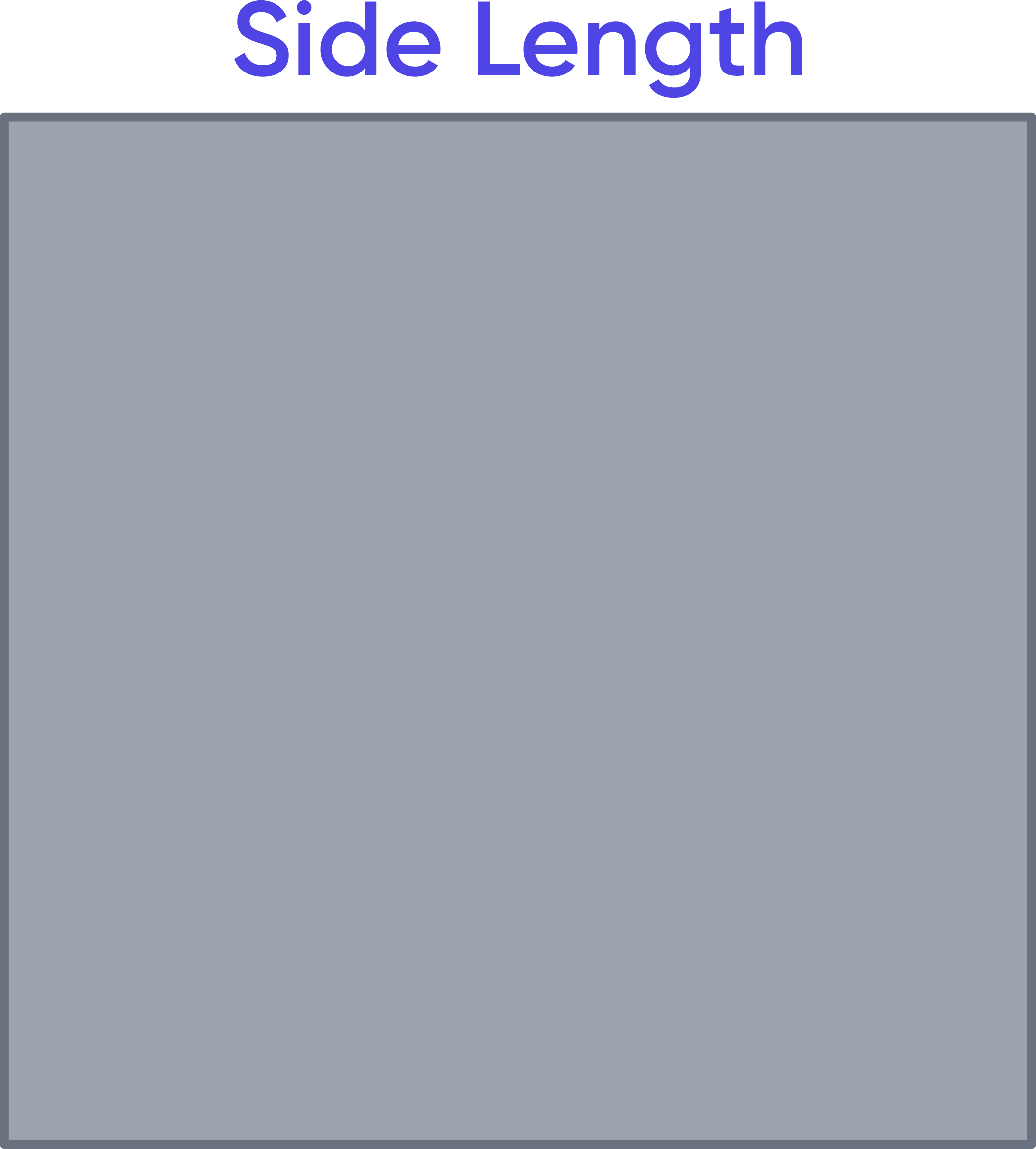The side length is a. Then, $$A = a^2$$ ## Rectangle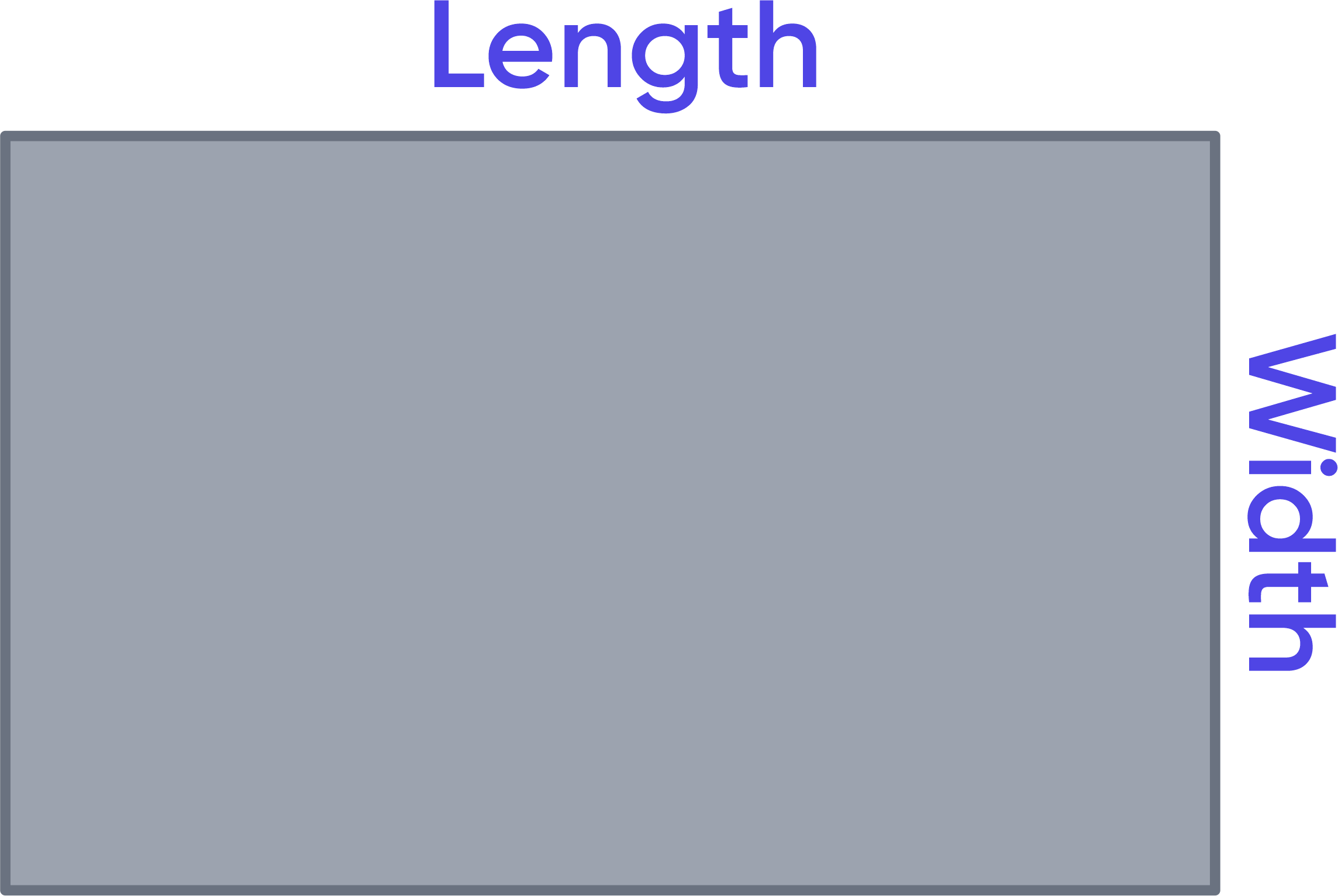Let a be the length and b be the width. Then, $$A = a × b$$ ## Rectangle border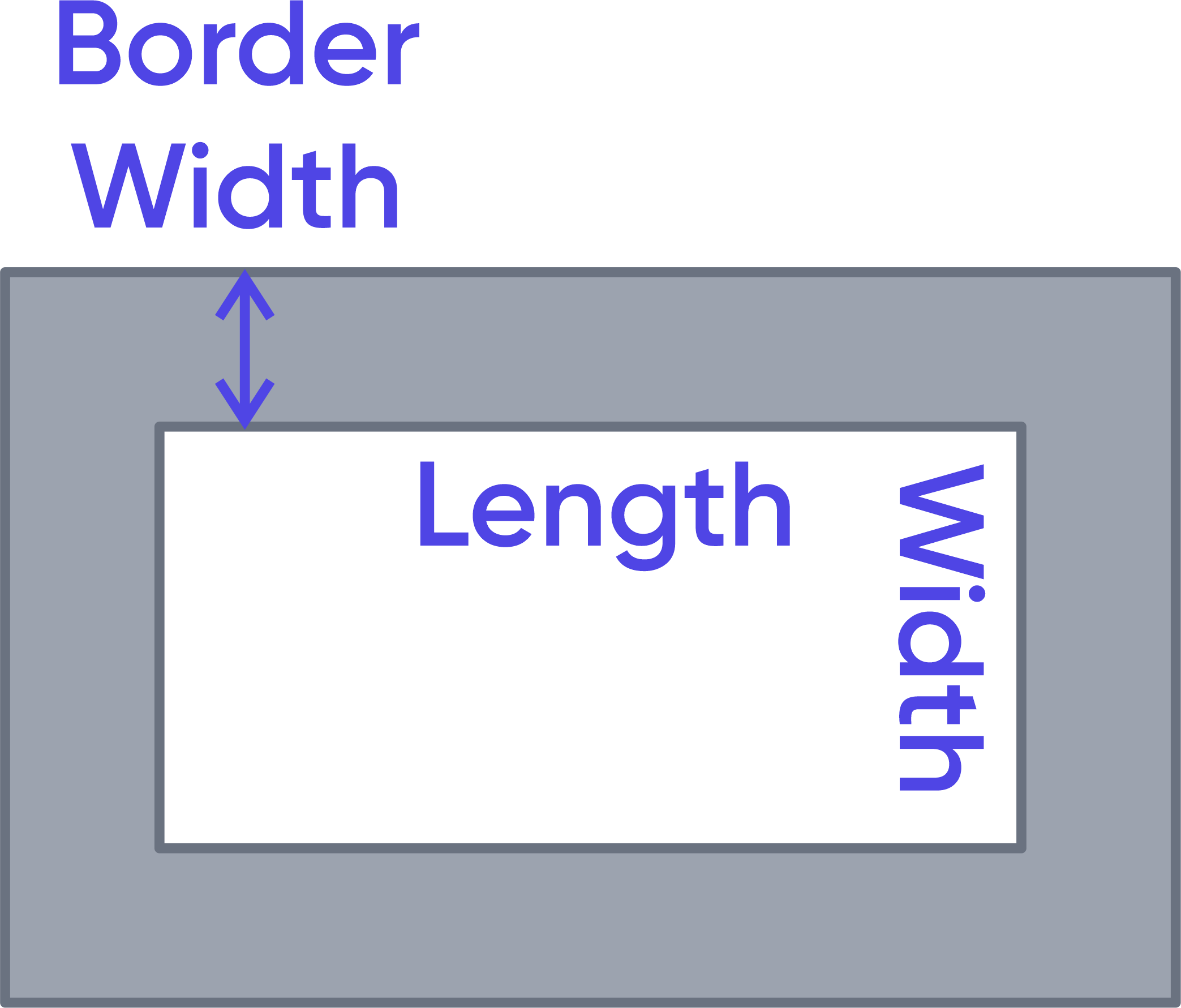Let a be the length, b be the width, and c be the border width. The square footage of the border area can be calculated as the difference between the square footage of the total area and the square footage of the inner area: $$Inner\ Area=A_1=a×b$$ $$Total\ Area=A_2=(a+2c)(b+2c)$$ $$A=A_2-A_1$$ ## Circle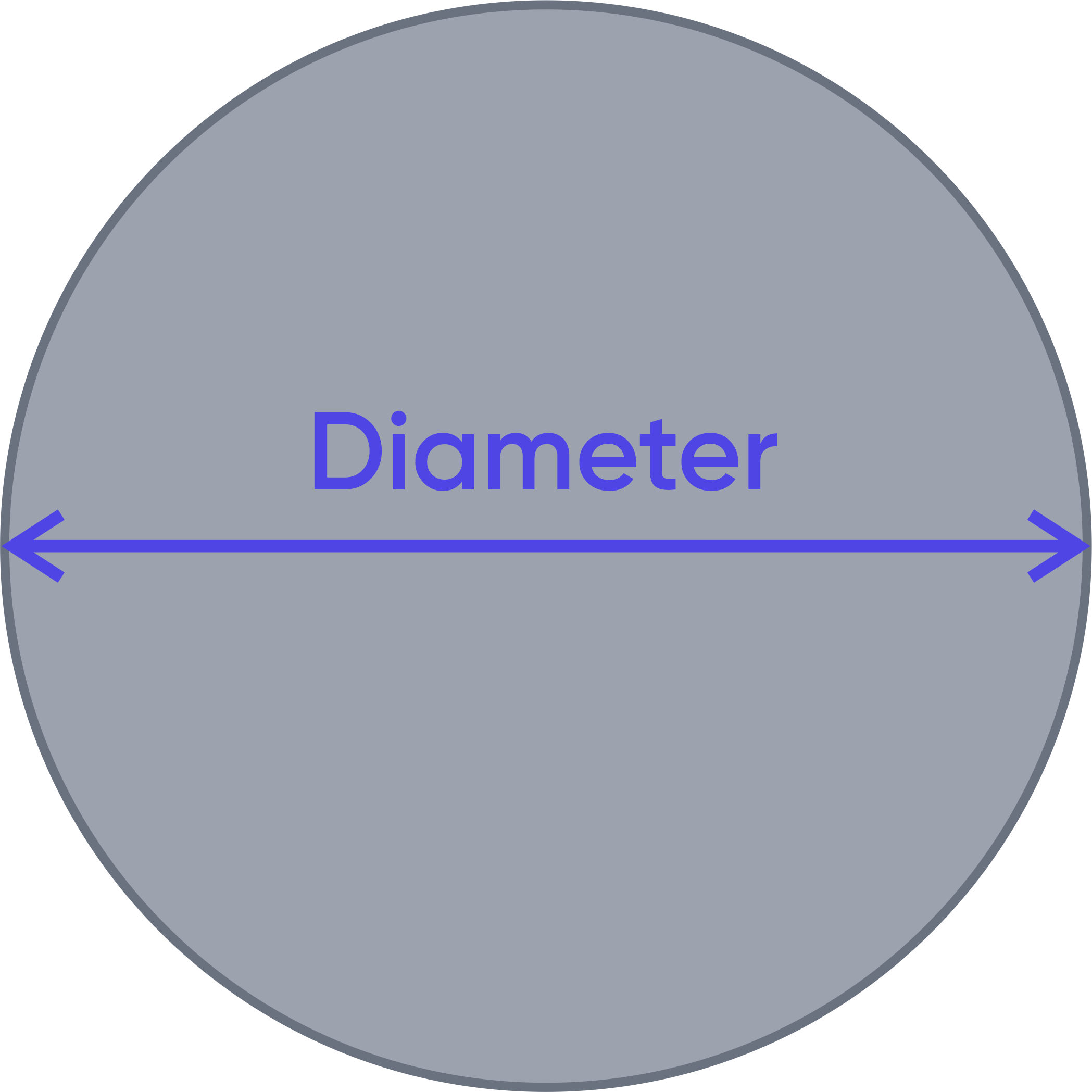Let the diameter equal d. Then, $$A=π\frac{d^2}{4}$$ or $$A=π r^2$$ ## Circle Border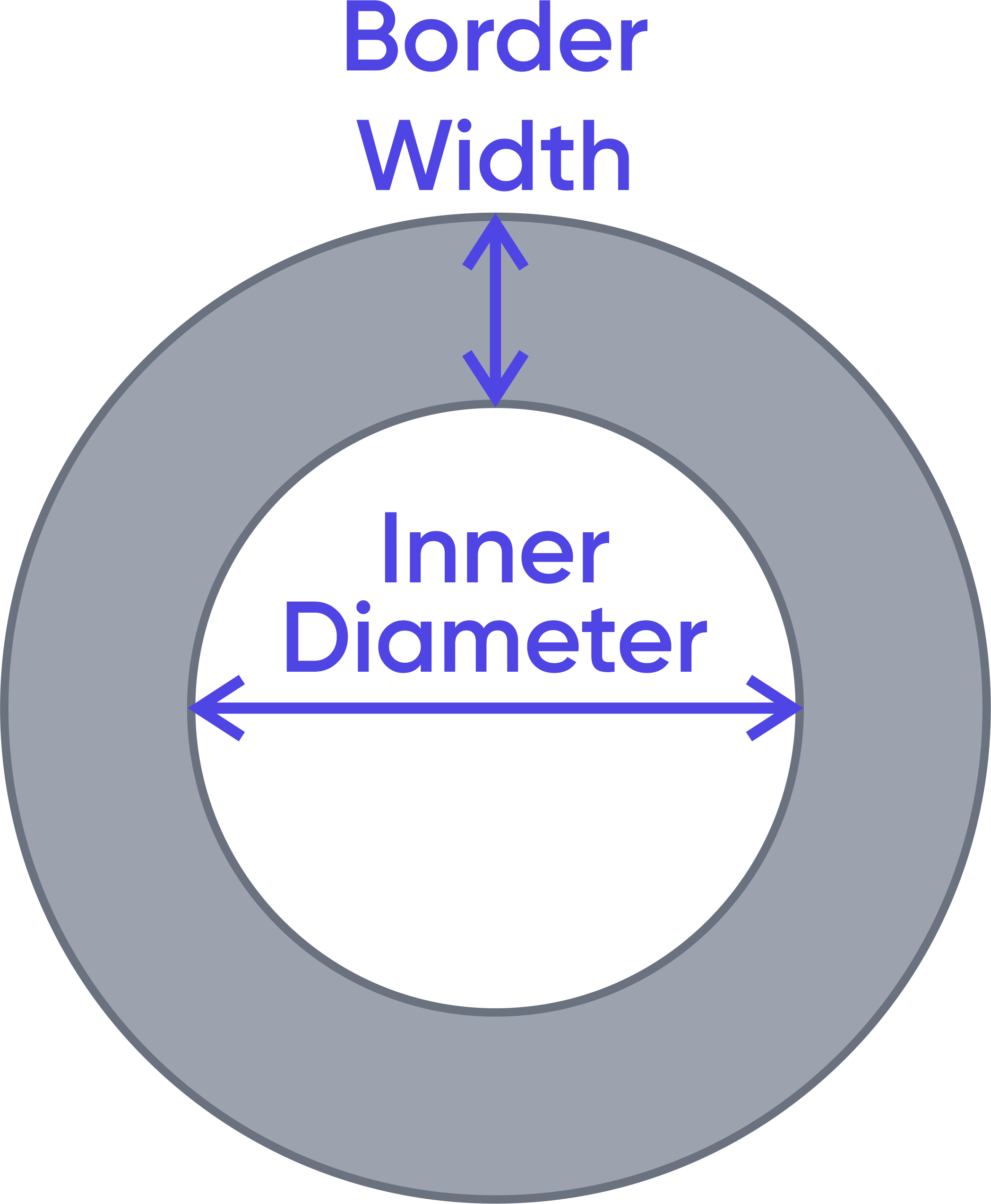Allow dᵢ for the inner diameter, dₒ for the outer diameter, and c for the border width. This calculation assumes that only the inner diameter dᵢ and border width c are known. The square footage of the border area can be calculated as the difference between the outer circle's square footage and the inner circle's square footage. We can find the outer diameter as follows: $$d_o=d_i+2c$$ Therefore, $$Inner\ Area=A_1=π\frac{{d_i}^2}{4}$$ $$Outer\ Area=A_2=π\frac{{d_o}^2}{4}$$ $$A=A_2-A_1$$ ## Annulus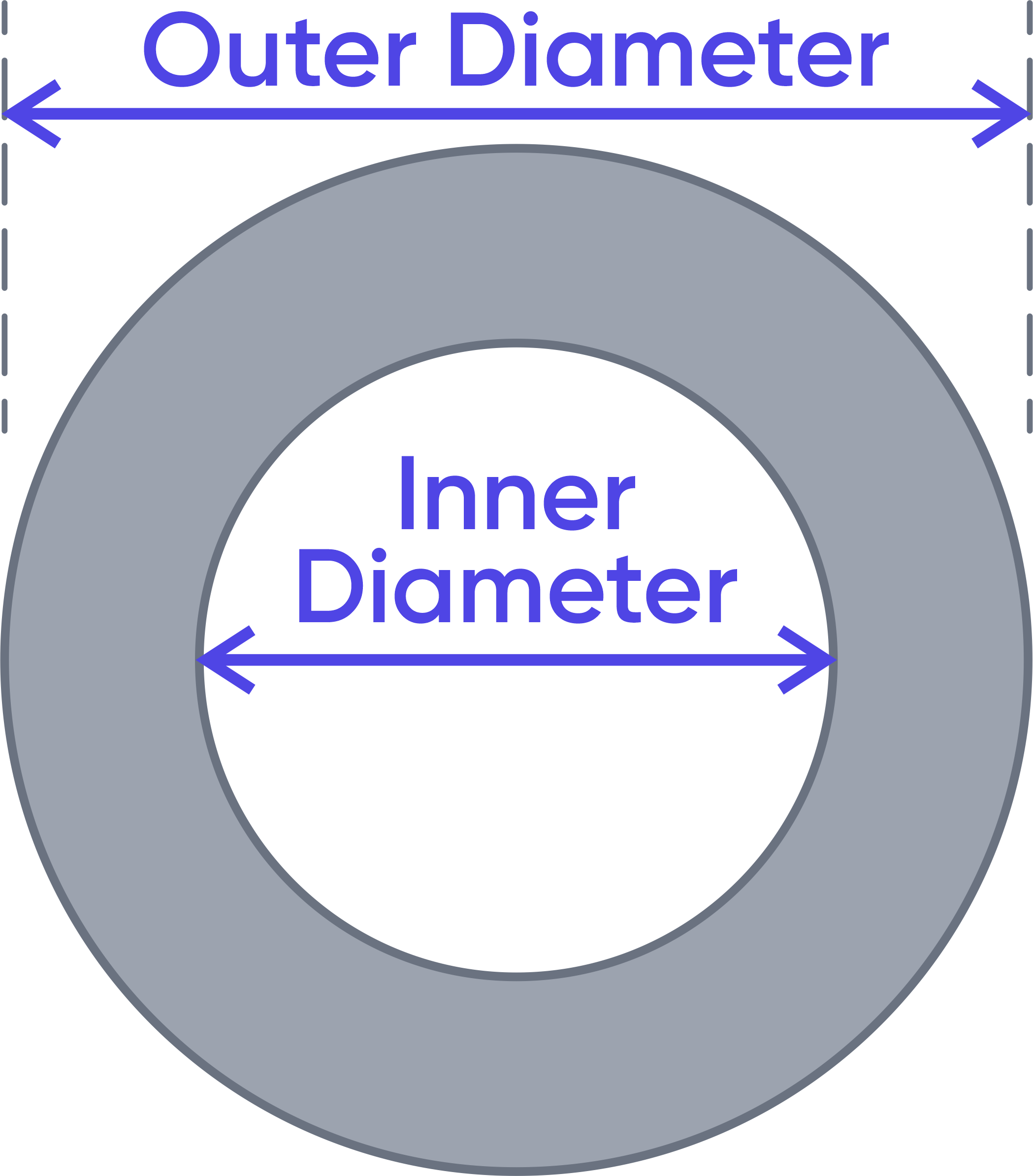This type of calculation refers to the same shape as the circle border type of calculation. In this case, we assume that the inner diameter dᵢ and the outer diameter dₒ are known. Similarly to the previous shape, $$Inner\ Area=A_1=π\frac{{d_i}^2}{4}$$ $$Outer\ Area=A_2=π\frac{{d_o}^2}{4}$$ $$A=A_2-A_1$$ ## Triangle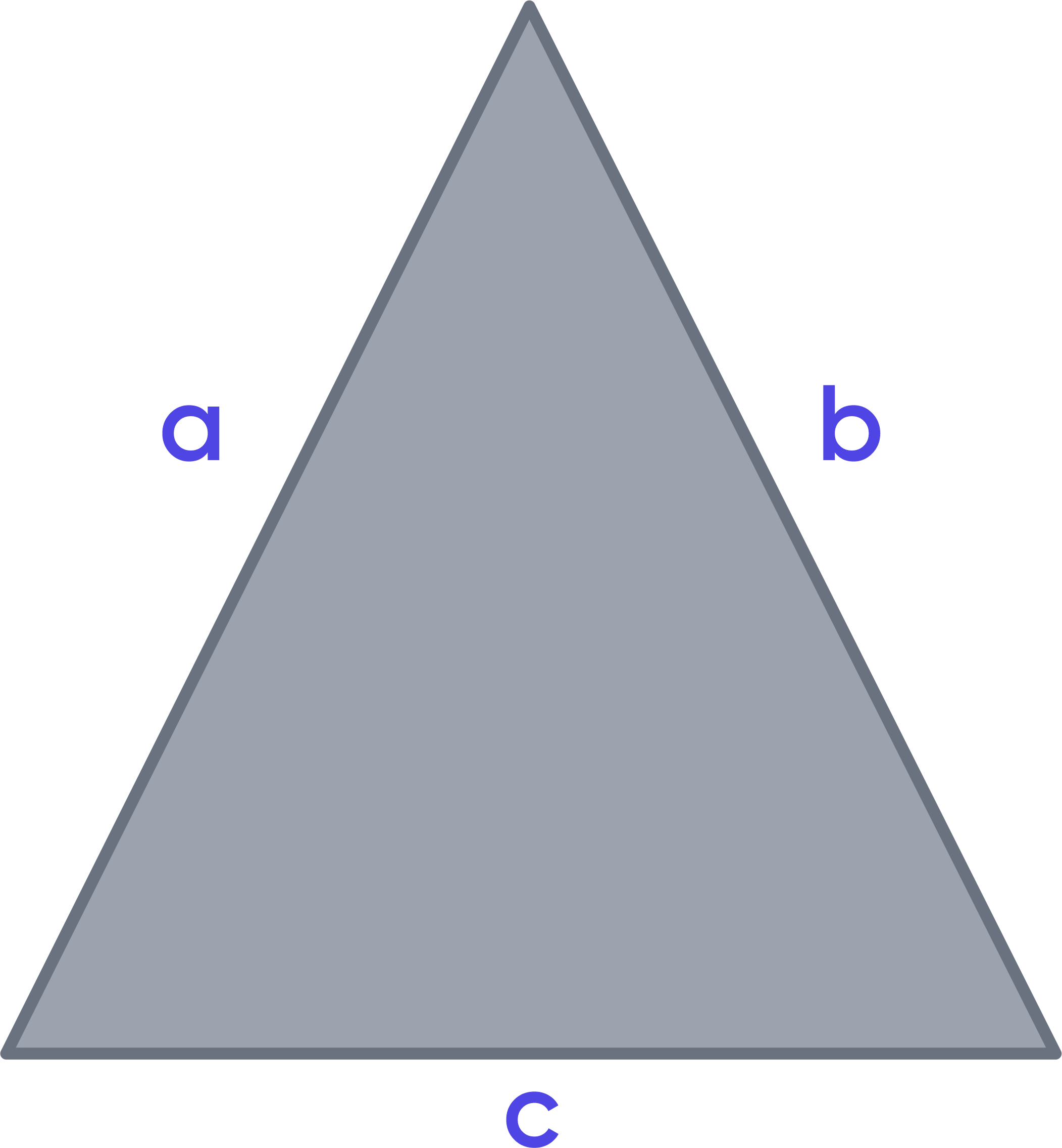Let the triangle's sides be a, b, and c. Then the area of this triangle can be found as follows: $$A=\sqrt{s\left(s-a\right)\left(s-b\right)\left(s-c\right)}$$ where $$s=\frac{a+b+c}{2}$$ ## Trapezoid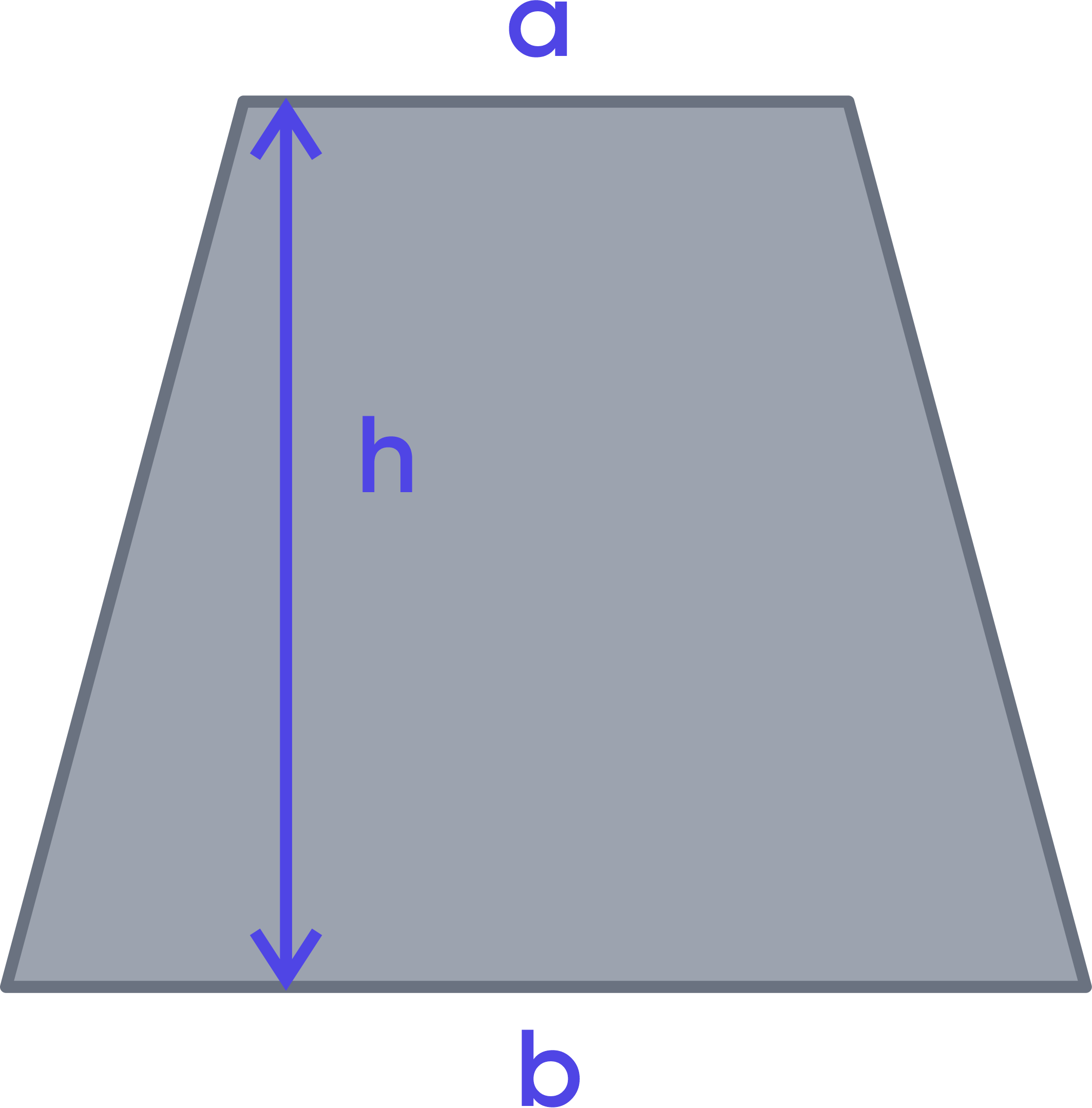Let a trapezoid's bases be a and b, and its altitude be h. Then the area of the trapezoid can be found as follows: $$A=\frac{a+b}{2}× h$$ ## Practical example Let's assume we need to find the square footage of a rectangular room and the costs associated with covering the floor of this room with wooden tiles. First, we will have to measure the room's length and width. Let's assume that the room's length is 15 feet, and the room's width is 9 feet. We also know the necessary tiles are sold at$86 per square foot.

To perform the calculations, we first need to choose "Rectangle" from the list of area shapes drop-down. Then we have to input the values of Length = 15 and Width = 9 and set the corresponding units to "ft" for both inputs. Since we only consider one room, we leave the quantity at 1. As the last step, we enter the price of $86 per square foot in the relevant field of the "Optional Cost Calculation." Then we press "Calculate." The calculator provides us with the following answer: • square footage = 135 ft², • square inches = 19,440 in², • square yards = 15 yd², • square meters = 12.54 m², • acres = 0.00310 acre, • cost =$ 11,610.